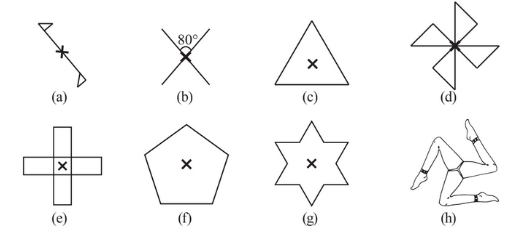# 2. Give the order of rotational symmetry for each figure:(a) The given figure has rotational symmetry aboutso it has ordered as 2.

(b) The given figure has rotational symmetry aboutso it has ordered as 2.

(c) The given figure has rotational symmetry aboutso it has ordered as 3.

(d) The given figure has rotational symmetry aboutso it has ordered as 4.

(e) The given figure has rotational symmetry aboutso it has ordered as 4.

(f) The given figure has rotational symmetry aboutso it has ordered as 5.

(g) The given figure has rotational symmetry aboutso it has ordered as 6.

(h) The given figure has rotational symmetry aboutso it has ordered as 3.

## Related Chapters

### Preparation Products

##### JEE Main Rank Booster 2021

This course will help student to be better prepared and study in the right direction for JEE Main..

₹ 13999/- ₹ 9999/-
##### Rank Booster NEET 2021

This course will help student to be better prepared and study in the right direction for NEET..

₹ 13999/- ₹ 9999/-
##### Knockout JEE Main April 2021 (Easy Installments)

An exhaustive E-learning program for the complete preparation of JEE Main..

₹ 4999/-
##### Knockout NEET May 2021

An exhaustive E-learning program for the complete preparation of NEET..

₹ 22999/- ₹ 14999/-